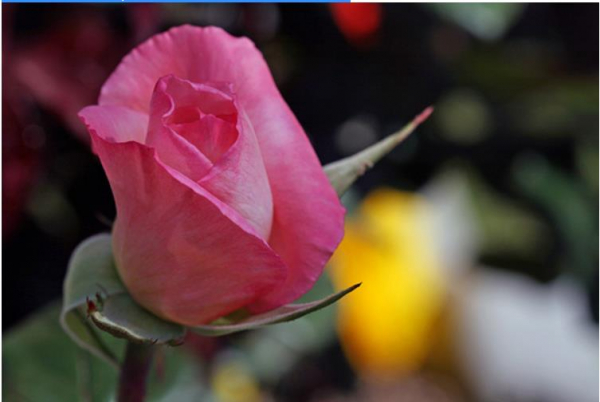# How can Tensorflow be used to download flower dataset into the environment?

The flower dataset can be downloaded using a google API that basically links to the flower dataset. The ‘get_file’ method can be used to pass the API as a parameter. Once this is done, the data gets downloaded into the environment.

We will be using the flowers dataset, which contains images of several thousands of flowers. It contains 5 sub-directories, and there is one sub-directory for every class.

We are using the Google Colaboratory to run the below code. Google Colab or Colaboratory helps run Python code over the browser and requires zero configuration and free access to GPUs (Graphical Processing Units). Colaboratory has been built on top of Jupyter Notebook.

import numpy as np
import os
import PIL
import PIL.Image
import tensorflow as tf
import tensorflow_datasets as tfds
print("The Tensorflow version is :")
print(tf.__version__)

import pathlib
data_dir = tf.keras.utils.get_file(origin=dataset_url,
fname='flower_photos',
untar=True)
data_dir = pathlib.Path(data_dir)

print("The number of images in the dataset")
image_count = len(list(data_dir.glob('*/*.jpg')))
print(image_count)

print("Sample data in the dataset")
roses = list(data_dir.glob('roses/*'))
PIL.Image.open(str(roses))

print("Some more sample data from the dataset")
roses = list(data_dir.glob('roses/*'))
PIL.Image.open(str(roses))

## Output

The Tensorflow version is :
2.4.0
228818944/228813984 [==============================] - 4s 0us/step
The number of images in the dataset
3670
Sample data in the dataset
Some more sample data from the dataset